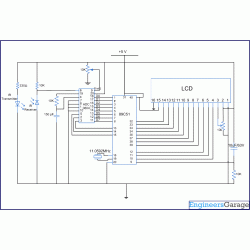# Distance measurement using InfraRed sensor with ADC0804 & 8051 microcontroller (AT89C51)

Posted by Unknown at 9:25 PM
Infrared sensors find numerous applications in electronic systems. Commonly used as obstacle detector, their output is used in digital form (high & low logic) by employing a comparator.  This topic explains a way to use the sensor’s output in its original analog form. Thus, along with detecting an obstacle, its exact distance can also be obtained. This is achieved by processing the output of IR sensor through an ADC0804 (analog to digital converter). The ADC is calibrated to get almost accurate distance measurement.

The measured distance is also displayed on an LCD screen. The ADC0804 and LCD are interfaced with 8051 microcontroller (AT89C51) to perform these operations. The major drawback of IR based sensors is their capability of detecting short distances.

DESCRIBTION:
• This project mainly consists of three units: a sensor unit, an ADC component and the LCD module.

IR LED. The output voltage level of this IR sensor depends upon the intensity of IR rays received by the receiver. The intensity, in turn, depends on the distance between the sensor module and the obstacle. When the distance between IR pair and obstacle is lesser, more IR radiations fall on the receiver, and vice versa. The receiver along with a resistor forms a voltage divider whose output is supplied as the input for ADC0804.

CIRCUIT DIAGRAM:
•CODE:
• ``````
//Program to interface IR sensor using ADC 0804. Set Vref =1.5v for ADC 0804

#include<reg51.h>

#define port P3

#define dataport P0

#define sec 100

sbit rs = port^0;

sbit rw = port^1;

sbit e = port^2;

sbit wr= port^3;

sbit rd= port^4;

sbit intr= port^5;

int test_final=0 ,shift=0;

void delay(unsigned int msec ) // Time delay function

{

int i ,j ;

for(i=0;i<msec;i++)

for(j=0; j<1275; j++);

}

void lcd_cmd(unsigned char item) // Function to send command to LCD

{

dataport = item;

rs= 0;

rw=0;

e=1;

delay(1);

e=0;

return;

}

void lcd_data(unsigned char item)  // Function to send data to LCD

{

dataport = item;

rs= 1;

rw=0;

e=1;

delay(1);

e=0;

return;

}

void lcd_data_string(unsigned char *str) // Function to send string to LCD

{

int i=0;

while(str[i]!='\0')

{

lcd_data(str[i]);

i++;

delay(1);

}

return;

}

void convert()

{

int s;

lcd_cmd(0x81);

delay(2);

lcd_data_string("output:");

s=test_final/100;

test_final=test_final%100;

lcd_cmd(0x8a);

if(s!=0)

lcd_data(s+48);

else

lcd_cmd(0x06);

s=test_final/10;

test_final=test_final%10;

lcd_data(s+48);

lcd_data(test_final+48);

lcd_data(' ');

if(shift>16)

{

lcd_cmd(0xc0+(shift-1));

lcd_data_string("       ");

shift=0;

}

lcd_cmd(0xc0+(shift-1));

lcd_data(' ');

lcd_cmd(0xc0+shift);

lcd_data_string("CALIBRATE IT");

delay(30);

}

void main()

{

lcd_cmd(0x38);  //2 Line, 5X7 Matrix

delay(2);

lcd_cmd(0x01);  // Clear Screen

delay(2);

lcd_cmd(0x81);  // Setting cursor to first position of first line

delay(2);

while(1)

{

shift++;

delay(1);

rd=1;

wr=0;

delay(1);

wr=1;

while(intr==1);

rd=0;

delay(1);

intr=1;

convert();

}

}
```
```
COMPONENTS:
• IR LED | Infrared LED
• AT89C51 Microcontroller
• Preset
• LCD
Tags:

1.Is this the code for the entire project..??

thnx for sharing..

2.Really thank for the code

3.really Thanks for the code. I must go for a trail.

THANKS FOR UR COMMENT ....

## Disclaimer

This blogs is an effort to club the scattered information about engineering and project titles and ideas available in the web. While every effort is made to ensure the accuracy of the information on this site, no liability is accepted for any consequences of using it. Most of the material and information are taken from other blogs and site with the help of search engines. If any posts here are hitting the copyrights of any publishers, kindly mail the details to educations360@gmail.com It will be removed immediately.

## Alexa Rank